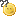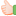# Thread: Firmware v1.9.2.7 CR3c [Oleg]

1.Junior Member
Join Date
Feb 2005
Posts
13
I have a problem with this firmware. It looks like that router doesn't connect to time.nist.gov (there is nothing in the log that says that it even tried to connect), so my time in the router is: Fri, 02 Jan 1970.

Another problem is the log:
Code:
```Jan  2 05:08:34 kernel: DROPIN=ppp0 OUT= MAC= SRC=193.77.104.218 DST=193.77.85.194 LEN=48 TOS=0x00 PREC=0x00 TTL=124 ID=22543 DF PROTO=TCP SPT=2394 DPT=445 SEQ=1530047695 ACK=0 WINDOW=16384 RES=0x00 SYN URGP=0 OPT (0204058601010402)
Jan  2 05:08:35 kernel: DROPIN=ppp0 OUT= MAC= SRC=193.77.104.218 DST=193.77.85.194 LEN=48 TOS=0x00 PREC=0x00 TTL=124 ID=22545 DF PROTO=TCP SPT=2353 DPT=31337 SEQ=1526670527 ACK=0 WINDOW=16384 RES=0x00 SYN URGP=0 OPT (0204058601010402)
Jan  2 05:10:14 kernel: DROPIN=ppp0 OUT= MAC= SRC=193.77.50.193 DST=193.77.85.194 LEN=48 TOS=0x00 PREC=0x00 TTL=126 ID=8367 DF PROTO=TCP SPT=4320 DPT=445 SEQ=3019354163 ACK=0 WINDOW=64800 RES=0x00 SYN URGP=0 OPT (020405A001010402)
Jan  2 05:10:17 kernel: DROPIN=ppp0 OUT= MAC= SRC=193.77.50.193 DST=193.77.85.194 LEN=48 TOS=0x00 PREC=0x00 TTL=126 ID=8550 DF PROTO=TCP SPT=4320 DPT=445 SEQ=3019354163 ACK=0 WINDOW=64800 RES=0x00 SYN URGP=0 OPT (020405A001010402)
Jan  2 05:11:08 kernel: DROPIN=ppp0 OUT= MAC= SRC=193.77.50.193 DST=193.77.85.194 LEN=48 TOS=0x00 PREC=0x00 TTL=126 ID=12581 DF PROTO=TCP SPT=1999 DPT=445 SEQ=3111494455 ACK=0 WINDOW=64800 RES=0x00 SYN URGP=0 OPT (020405A001010402)
Jan  2 05:11:11 kernel: DROPIN=ppp0 OUT= MAC= SRC=193.77.50.193 DST=193.77.85.194 LEN=48 TOS=0x00 PREC=0x00 TTL=126 ID=12794 DF PROTO=TCP SPT=1999 DPT=445 SEQ=3111494455 ACK=0 WINDOW=64800 RES=0x00 SYN URGP=0 OPT (020405A001010402)
Jan  2 05:11:19 kernel: DROPIN=ppp0 OUT= MAC= SRC=194.165.127.182 DST=193.77.85.194 LEN=48 TOS=0x00 PREC=0x00 TTL=124 ID=4672 DF PROTO=TCP SPT=2864 DPT=445 SEQ=2690808095 ACK=0 WINDOW=16384 RES=0x00 SYN URGP=0 OPT (0204058601010402)
Jan  2 05:11:45 kernel: DROPIN=eth0 OUT= MAC=ff:ff:ff:ff:ff:ff:00:0a:e6:d0:cc:b4:08:00 SRC=0.0.0.0 DST=255.255.255.255 LEN=328 TOS=0x00 PREC=0x00 TTL=128 ID=2871 PROTO=UDP SPT=68 DPT=67 LEN=308
Jan  2 05:11:49 kernel: DROPIN=ppp0 OUT= MAC= SRC=193.77.230.16 DST=193.77.85.194 LEN=48 TOS=0x00 PREC=0x00 TTL=126 ID=63996 DF PROTO=TCP SPT=3848 DPT=139 SEQ=2361238481 ACK=0 WINDOW=16384 RES=0x00 SYN URGP=0 OPT (0204058601010402)
Jan  2 05:11:49 kernel: DROPIN=eth0 OUT= MAC=ff:ff:ff:ff:ff:ff:00:0a:e6:d0:cc:b4:08:00 SRC=0.0.0.0 DST=255.255.255.255 LEN=328 TOS=0x00 PREC=0x00 TTL=128 ID=3034 PROTO=UDP SPT=68 DPT=67 LEN=308
Jan  2 05:11:52 kernel: DROPIN=ppp0 OUT= MAC= SRC=193.77.230.16 DST=193.77.85.194 LEN=48 TOS=0x00 PREC=0x00 TTL=126 ID=64100 DF PROTO=TCP SPT=3848 DPT=139 SEQ=2361238481 ACK=0 WINDOW=16384 RES=0x00 SYN URGP=0 OPT (0204058601010402)
Jan  2 05:11:58 kernel: DROPIN=eth0 OUT= MAC=ff:ff:ff:ff:ff:ff:00:0a:e6:d0:cc:b4:08:00 SRC=0.0.0.0 DST=255.255.255.255 LEN=328 TOS=0x00 PREC=0x00 TTL=128 ID=3476 PROTO=UDP SPT=68 DPT=67 LEN=308
Jan  2 05:12:14 kernel: DROPIN=eth0 OUT= MAC=ff:ff:ff:ff:ff:ff:00:0a:e6:d0:cc:b4:08:00 SRC=0.0.0.0 DST=255.255.255.255 LEN=328 TOS=0x00 PREC=0x00 TTL=128 ID=4155 PROTO=UDP SPT=68 DPT=67 LEN=308
Jan  2 05:12:20 kernel: DROPIN=ppp0 OUT= MAC= SRC=193.77.113.136 DST=193.77.85.194 LEN=48 TOS=0x00 PREC=0x00 TTL=126 ID=24045 DF PROTO=TCP SPT=4764 DPT=445 SEQ=739160563 ACK=0 WINDOW=64800 RES=0x00 SYN URGP=0 OPT (020405A001010402)
Jan  2 05:12:23 kernel: DROPIN=ppp0 OUT= MAC= SRC=193.77.113.136 DST=193.77.85.194 LEN=48 TOS=0x00 PREC=0x00 TTL=126 ID=24073 DF PROTO=TCP SPT=4764 DPT=445 SEQ=739160563 ACK=0 WINDOW=64800 RES=0x00 SYN URGP=0 OPT (020405A001010402)
Jan  2 05:13:02 kernel: DROPIN=ppp0 OUT= MAC= SRC=193.77.43.49 DST=193.77.85.194 LEN=48 TOS=0x00 PREC=0x00 TTL=124 ID=27391 DF PROTO=TCP SPT=4105 DPT=445 SEQ=4064038337 ACK=0 WINDOW=16384 RES=0x00 SYN URGP=0 OPT (0204058601010402)
Jan  2 05:13:04 kernel: DROPIN=ppp0 OUT= MAC= SRC=193.77.43.49 DST=193.77.85.194 LEN=48 TOS=0x00 PREC=0x00 TTL=124 ID=28111 DF PROTO=TCP SPT=4105 DPT=445 SEQ=4064038337 ACK=0 WINDOW=16384 RES=0x00 SYN URGP=0 OPT (0204058601010402)
Jan  2 05:13:20 kernel: DROPIN=ppp0 OUT= MAC= SRC=193.77.19.230 DST=193.77.85.194 LEN=48 TOS=0x00 PREC=0x00 TTL=124 ID=31832 DF PROTO=TCP SPT=4215 DPT=445 SEQ=2151228714 ACK=0 WINDOW=16384 RES=0x00 SYN URGP=0 OPT (020405A001010402)
Jan  2 05:13:23 kernel: DROPIN=ppp0 OUT= MAC= SRC=193.77.19.230 DST=193.77.85.194 LEN=48 TOS=0x00 PREC=0x00 TTL=124 ID=32156 DF PROTO=TCP SPT=4215 DPT=445 SEQ=2151228714 ACK=0 WINDOW=16384 RES=0x00 SYN URGP=0 OPT (020405A001010402)
Jan  2 05:14:05 kernel: DROPIN=ppp0 OUT= MAC= SRC=81.23.250.167 DST=193.77.85.194 LEN=62 TOS=0x00 PREC=0x00 TTL=58 ID=50807 DF PROTO=UDP SPT=4246 DPT=1035 LEN=42
Jan  2 05:14:05 kernel: DROPIN=ppp0 OUT= MAC= SRC=81.23.250.167 DST=193.77.85.194 LEN=99 TOS=0x00 PREC=0x00 TTL=58 ID=50817 DF PROTO=UDP SPT=4246 DPT=1035 LEN=79
Jan  2 05:15:13 kernel: DROPIN=ppp0 OUT= MAC= SRC=220.135.85.189 DST=193.77.85.194 LEN=40 TOS=0x00 PREC=0x00 TTL=113 ID=11791 PROTO=TCP SPT=4662 DPT=2604 SEQ=0 ACK=620502954 WINDOW=0 RES=0x00 ACK RST URGP=0
Jan  2 05:16:50 kernel: DROPIN=ppp0 OUT= MAC= SRC=80.203.82.175 DST=193.77.85.194 LEN=48 TOS=0x00 PREC=0x00 TTL=110 ID=19516 DF PROTO=TCP SPT=65239 DPT=6406 SEQ=3520080491 ACK=0 WINDOW=16384 RES=0x00 SYN URGP=0 OPT (020405B401010402)
Jan  2 05:16:53 kernel: DROPIN=ppp0 OUT= MAC= SRC=80.203.82.175 DST=193.77.85.194 LEN=48 TOS=0x00 PREC=0x00 TTL=110 ID=19626 DF PROTO=TCP SPT=65239 DPT=6406 SEQ=3520080491 ACK=0 WINDOW=16384 RES=0x00 SYN URGP=0 OPT (020405B401010402)
Jan  2 05:16:59 kernel: DROPIN=ppp0 OUT= MAC= SRC=80.203.82.175 DST=193.77.85.194 LEN=48 TOS=0x00 PREC=0x00 TTL=110 ID=19809 DF PROTO=TCP SPT=65239 DPT=6406 SEQ=3520080491 ACK=0 WINDOW=16384 RES=0x00 SYN URGP=0 OPT (020405B401010402)
Jan  2 05:17:29 kernel: DROPIN=eth0 OUT= MAC=ff:ff:ff:ff:ff:ff:00:0a:e6:d0:cc:b4:08:00 SRC=0.0.0.0 DST=255.255.255.255 LEN=328 TOS=0x00 PREC=0x00 TTL=128 ID=17587 PROTO=UDP SPT=68 DPT=67 LEN=308
Jan  2 05:17:33 kernel: DROPIN=eth0 OUT= MAC=ff:ff:ff:ff:ff:ff:00:0a:e6:d0:cc:b4:08:00 SRC=0.0.0.0 DST=255.255.255.255 LEN=328 TOS=0x00 PREC=0x00 TTL=128 ID=17773 PROTO=UDP SPT=68 DPT=67 LEN=308
Jan  2 05:17:40 kernel: DROPIN=eth0 OUT= MAC=ff:ff:ff:ff:ff:ff:00:0a:e6:d0:cc:b4:08:00 SRC=0.0.0.0 DST=255.255.255.255 LEN=328 TOS=0x00 PREC=0x00 TTL=128 ID=18076 PROTO=UDP SPT=68 DPT=67 LEN=308
Jan  2 05:17:56 kernel: DROPIN=eth0 OUT= MAC=ff:ff:ff:ff:ff:ff:00:0a:e6:d0:cc:b4:08:00 SRC=0.0.0.0 DST=255.255.255.255 LEN=328 TOS=0x00 PREC=0x00 TTL=128 ID=18622 PROTO=UDP SPT=68 DPT=67 LEN=308
...
...
...```
WHAT IS THIS???

I didn't get messages like this with the 1.8.2.1 firmware.2.Administrator
Join Date
Dec 2003
Location
Russian Federation
Posts
8,353Originally Posted by dejay
WHAT IS THIS???

I didn't get messages like this with the 1.8.2.1 firmware.
This is your firewall log.3.Member
Join Date
May 2004
Location
Moscow, Russia
Posts
50Originally Posted by Oleg
This is your firewall log.
I beileve that space was missed after DROP in sources, and DROPIN is a bit cryptic IMHO.

So it goes.
Roofcat4.Junior Member
Join Date
Jan 2005
Posts
7Originally Posted by dejay
I have a problem with this firmware. It looks like that router doesn't connect to time.nist.gov (there is nothing in the log that says that it even tried to connect), so my time in the router is: Fri, 02 Jan 1970.
I had the same problem. After I changed time.nist.gov to time-a.nist.gov it worked fine again.5.Member
Join Date
May 2004
Location
Moscow, Russia
Posts
50
Looks like it was a problem with time.nist.gov last weekend. Since 3 am monday it returned to normal (at least as I see it).

So it goes.
Roofcat6.Originally Posted by RoofCat
Looks like it was a problem with time.nist.gov last weekend. Since 3 am monday it returned to normal (at least as I see it).

So it goes.
Roofcat
Yes had also some problems with this time server....7.Registered User
Join Date
Mar 2005
Posts
3

## Client mode on WL-500bv2

Hi,
i have first time problem with Client mode at ASUS. I have WL-500bv2 and I upload the firmware WL500bv2-1.9.2.7-3c.trx. But after login to web interface and go to Wireless>Advanced, then don't show the settings for client mode, only items: Hide SSID, Fragmentation Threshold, RTS Threshold, Beacon Interval.
In the Admin web console after command "nvram get nobr" I become answer "1" > is Ralink, but after command "wl status" I become answer "wl driver adapter not found".
I have total 4 ASUS in client mode set up, but this one with v2 modification doesn't work. Can you help me, please?8.Administrator
Join Date
Dec 2003
Location
Russian Federation
Posts
8,353Originally Posted by a3xx
I have total 4 ASUS in client mode set up, but this one with v2 modification doesn't work. Can you help me, please?
wl500bv2 units are not supported.9.Registered User
Join Date
Mar 2005
Posts
3

##Originally Posted by Oleg
wl500bv2 units are not supported.
Thanks, I changed it for WL-500g and works perfect.10.Administrator
Join Date
Dec 2003
Location
Russian Federation
Posts
8,353Originally Posted by Colonizer
if i connect directly to my ISP without the Asus, i did not get any DNS Server Errors, i can connect to Ebay in my account.
with the CR3c i was not able to log in ebay.

strange
maybe ill tray again next week
Please check your PM.11.Member
Join Date
Apr 2004
Posts
70
Hello!

How can I setup PPTP and get WAN-IP from DHCP?####Posting Permissions

• You may not post new threads
• You may not post replies
• You may not post attachments
• You may not edit your posts
•